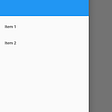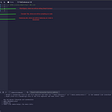# Anatomy of a Stack Runtime

`object "ContractA" {    code {        datacopy(0, dataoffset("Runtime"), datasize("Runtime"))        return(0, datasize("Runtime"))    }    object "Runtime" {        code {            let _calldata := 256            let _output_pointer := 0                        // Copy calldata at the _calldata pointer            calldatacopy(_calldata, 0, calldatasize())                        // Read the first 4 bytes representing             // the signature of the called function            // https://solidity.readthedocs.io/en/v0.6.4/abi-spec.html#function-selector            let fn_sig := mslice(_calldata, 4)                        switch fn_sig            case 0xffffffff {                // do SOME COMPUTING                let result := 10                let result_length := 32                mstore(_output_pointer, result)                return(_output_pointer, result_length)            }            // other cases/function signatures            default {                // default case - revert with error code                mslicestore(_output_pointer, 0xeee1, 2)                revert(_output_pointer, 2)            }                        function mslice(position, length) -> result {                result := div(mload(position), exp(2, sub(256, mul(length, 8))))            }                        function mslicestore(_ptr, val, length) {                let slot := 32                mstore(_ptr, shl(mul(sub(slot, length), 8), val))            }        }    }}`
`f(some_bytes) => some_other_bytes`
`parse(some_bytes) => T1parse(some_other_bytes) => T2f(T1) => T2`
`x1 := new(uint(8))x2 := new(byte(32))`
`sig1 := signature(signature(uint), signature(8))sig2 := signature(signature(byte), signature(32))`

# Calling a function from another function: `apply`

`apply(T1) => T2T1 = Tuple(signature(f), input)T2 = f(input)apply(signature(f), input) = f(input)`
`object "ContractB" {    code {        datacopy(0, dataoffset("Runtime"), datasize("Runtime"))        return(0, datasize("Runtime"))    }    object "Runtime" {        code {            let _calldata := 256            let _output_pointer := 0                        calldatacopy(_calldata, 0, calldatasize())                        let fn_sig := mslice(_calldata, 4)                        switch fn_sig            case 0xffffffff {                let internal_fn_sig := mslice(add(_calldata, 4), 4)                let input_pointer := add(_calldata, 8)                                    let result_length := dtapply(                    internal_fn_sig,                    input_pointer,                    _output_pointer                )                return (_output_pointer, result_length)            }            // other cases/function signatures            default {                mslicestore(_output_pointer, 0xeee1, 2)                revert(_output_pointer, 2)            }                    function dtapply(                fsig,                input,                output_pointer            ) -> result_length            {                switch fsig                case 0xeeeeeeee {                    let a := mload(input)                    mstore(output_pointer, add(a, 2))                    result_length := 32                }                case 0xdddddddd {                    let a := mload(input)                    mstore(output_pointer, sub(a, 2))                    result_length := 32                }                // other cases/function signatures                default {                    // default case - revert with error code                    mslicestore(output_pointer, 0xeee2, 2)                    revert(output_pointer, 2)                }            }                        function mslice(position, length) -> result {                result := div(                    mload(position),                    exp(2, sub(256, mul(length, 8)))                )            }                        function mslicestore(_ptr, val, length) {                let slot := 32                mstore(_ptr, shl(mul(sub(slot, length), 8), val))            }        }    }}`

## Functional interaction for `apply`

`external Environment->Input Pointer:calldataInput Pointer->Type Interpreter:type(calldata[4:end])Type Interpreter->Parser:new T1 = parse(calldata[8:end], sig(T1))Parser->Type Interpreter:T1Type Interpreter->Type Interpreter:isFunction(T1)note over Type Interpreter:if TRUEType Interpreter->Function Call:apply(T1)Function Call->Type Interpreter:T2Type Interpreter->Output Pointer:set(T2)note over Type Interpreter:if FALSEType Interpreter->Output Pointer:set(T1)Output Pointer->external Environment:return(pointer_contents)`

# Recursive `apply`

`object "ContractB" {    code {        datacopy(0, dataoffset("Runtime"), datasize("Runtime"))        return(0, datasize("Runtime"))    }    object "Runtime" {        code {            let _calldata := 256            let _output_pointer := 0                        calldatacopy(_calldata, 0, calldatasize())                        let fn_sig := mslice(_calldata, 4)                        switch fn_sig            case 0xffffffff {                let internal_fn_sig := mslice(add(_calldata, 4), 4)                let input_pointer := add(_calldata, 8)                                    let result_length := dtapplyRecursive(                    internal_fn_sig,                    input_pointer,                    _output_pointer                )                return (_output_pointer, result_length)            }            // other cases/function signatures            default {                mslicestore(_output_pointer, 0xeee1, 2)                revert(_output_pointer, 2)            }                    function dtapplyRecursive(                fsig,                input,                output_pointer            ) -> result_length {                switch fsig                case 0xeeeeeeee {                    let a := mload(input)                    mstore(output_pointer, add(a, 2))                    result_length := 32                }                case 0xdddddddd {                    let a := mload(input)                    mstore(output_pointer, sub(a, 2))                    result_length := 32                }                case 0xcccccccc {                    let internal_fsig := mslice(input, 4)                    // skip the 4 byte signature & the 32 byte value                    let count := mslice(add(input, 36), 4)                                        let temporary_ptr := add(input, 4)                                        for { let i := 0 } lt(i, count) { i := add(i, 1) } {                        result_length := dtapplyRecursive(                            internal_fsig,                            temporary_ptr,                            output_pointer                        )                        temporary_ptr := output_pointer                    }                }                // other cases/function signatures                default {                    // default case - revert with error code                    mslicestore(output_pointer, 0xeee2, 2)                    revert(output_pointer, 2)                }            }                        function mslice(position, length) -> result {                result := div(                    mload(position),                    exp(2, sub(256, mul(length, 8)))                )            }                        function mslicestore(_ptr, val, length) {                let slot := 32                mstore(_ptr, shl(mul(sub(slot, length), 8), val))            }        }    }}`

# Next: Step 2

--

--

--

## More from Loredana Cirstea

Building bricks for the World Computer #ethereum #Pipeline #dType #EIP1900 https://github.com/loredanacirstea, https://www.youtube.com/c/LoredanaCirstea

Love podcasts or audiobooks? Learn on the go with our new app.

## Deploying Flutter Apps with Github Actions## Detect Flutter Drawer Open and Close## How to Convert HTML to DOCX in PHP## Code comments as a work of art## Comparison Among 3 Approaches to Convert a Web application into a Desktop application using…## Coding Challenge — Scrabble Help## Using Zomato API to fetch Restaurant data## How to Fix the Error “Record(s) cannot be read; no read permission” in Access## Loredana Cirstea

Building bricks for the World Computer #ethereum #Pipeline #dType #EIP1900 https://github.com/loredanacirstea, https://www.youtube.com/c/LoredanaCirstea

## Deploy: StackPath’s web application firewall to protect your Solana RPC## Structure & Building Blocks of Smart Contract## Learn Solidity — 2: Addresses and Global Variables